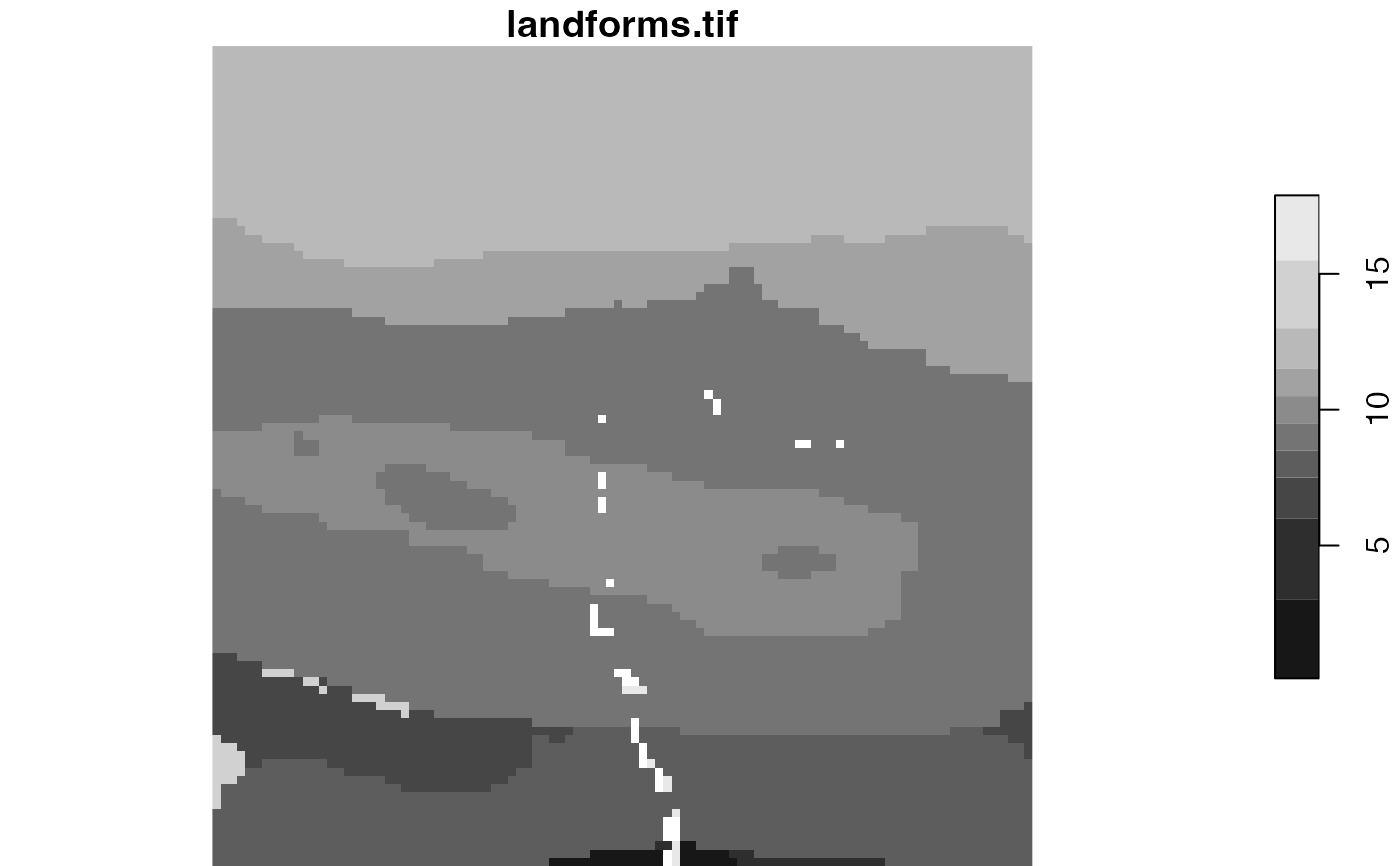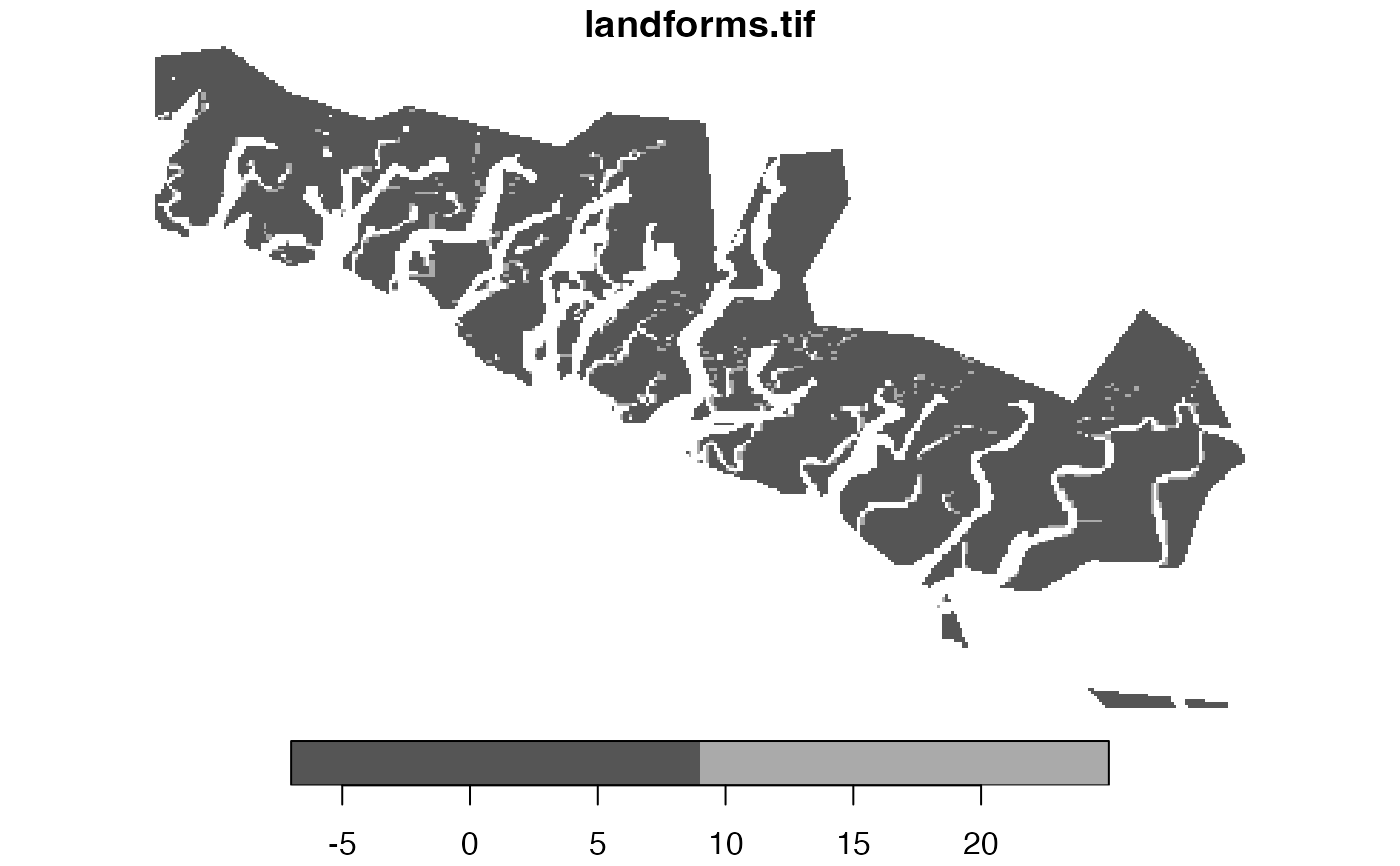Extracts a local landscape from categorical raster data based on its id and provided window argument.

lsp_extract(x, window, id, output = "stars")

## Arguments

x

Object of class stars, stars_proxy, or terra's SpatRaster.

window

Specifies areas for analysis. It can be either: NULL, a numeric value, or an sf object.

id

Id of the local landscape - it is possible to find in the output of lsp_signature(), lsp_search(), lsp_compare(), or lsp_add_clusters().

output

The class of the output. Either "stars" or terra

## Value

A starsor terra object cropped to the extent of a selected local landscape

## Examples

library(stars)
landform = read_stars(system.file("raster/landforms.tif", package = "motif"))
ecoregions = read_sf(system.file("vector/ecoregionss.gpkg", package = "motif"))

extract1 = lsp_extract(x = landform, window = 100, id = 25)
plot(extract1)ecoregions = st_transform(ecoregions, st_crs(landform))
extract2 = lsp_extract(x = landform, window = ecoregions["id"], id = 11)
plot(extract2)# \donttest{
# larger data example
library(stars)
landform = read_stars(system.file("raster/landform.tif", package = "motif"))
ecoregions = read_sf(system.file("vector/ecoregions.gpkg", package = "motif"))

extract1 = lsp_extract(x = landform, window = 100, id = 1895)
plot(extract1)
#> Error in image.stars(x, ..., axes = axes, breaks = breaks, col = col,     key.pos = key.pos, key.width = key.width, key.length = key.length,     main = NULL): rgb plotting not supported on this device

ecoregions = st_transform(ecoregions, st_crs(landform))
extract2 = lsp_extract(x = landform, window = ecoregions["id"], id = 7)
plot(extract2)#> Error in image.stars(x, ..., axes = axes, breaks = breaks, col = col,     key.pos = key.pos, key.width = key.width, key.length = key.length,     main = NULL): rgb plotting not supported on this device
# }